﻿ Measuring the Uncertainty of Human Reasoning

### Measuring the Uncertainty of Human Reasoning

Michael Gr. VoskoglouOPEN ACCESSPEER-REVIEWED

## Measuring the Uncertainty of Human Reasoning

Michael Gr. VoskoglouSchool of Technological Applications, Graduate Technological Educational Institute (T. E. I.) of Western Greece, Patras, Greece

### Abstract

Human reasoning is characterized by a degree of fuzziness and uncertainty. In the present paper we develop a fuzzy model for a better description of the reasoning process and we use the fuzzy systems’ total possibilistic uncertainty as well as the classical ’s entropy (properly modified for use in fuzzy environments) in measuring the individuals’ reasoning skills. Classroom experiments are also provided illustrating our results in practice.

### At a glance: Figures

1
Prev Next

• Voskoglou, Michael Gr.. "Measuring the Uncertainty of Human Reasoning." American Journal of Applied Mathematics and Statistics 2.1 (2014): 1-6.
• Voskoglou, M. G. (2014). Measuring the Uncertainty of Human Reasoning. American Journal of Applied Mathematics and Statistics, 2(1), 1-6.
• Voskoglou, Michael Gr.. "Measuring the Uncertainty of Human Reasoning." American Journal of Applied Mathematics and Statistics 2, no. 1 (2014): 1-6.

 Import into BibTeX Import into EndNote Import into RefMan Import into RefWorks

### 1. Introduction

Conscious direction of attention towards an external object causes the object to be received by mind in sequence to perception, experience, feeling, understanding, explaining, knowing, and finally acting for meaningful description and analytical solution.

Reasoning is the most important human brain operation that leads to creative methodologies, algorithms and deductions giving way to sustainable research and development. The main stages of human reasoning for reaching to a solution of an existing problem (not necessarily mathematical) involve imagination, visualization and idea generations (, p. 340, or , section 4).

For any external object, whether it exists materialistically or not, human beings try to imagine its properties in their minds. This gives them the power of initializing their individual thinking domain with whole freedom in any direction. Imagination includes the setting up of a suitable hypothesis or a set of logical rules for the problem at hand.

The visualization stage is to defend the representative hypothesis and logical propositions. Humans typically use a variety of representations to defend their hypotheses including algorithms, graphs, diagrams, charts, figures, etc. In particular, the geometric configuration of the objects appearing through imagination is the most common among these representations. In fact, after an object comes into existence vaguely in mind, it is necessary to know its shape, which is related to geometry. It is essential that the geometric configuration of the phenomenon must be visualized in mind in some way, even though it may be a simplification under a set of assumptions.

On the basis of their hypotheses the individuals generate relevant ideas. The ideas begin to crystallize and they are expressed verbally by a native language to other individuals to get their criticisms, comments, suggestions and support for the betterment of the mental thinking and scientific achievement. Finally, all the conclusions must be expressed in a language, which can then be converted into universally used symbolic logic based on the principles of mathematics. We emphasize that whatever are the means of reasoning the scientific arguments are expressed verbally prior to any symbolic and mathematical abstractions.

It is possible to state that with Newtonian classical physics science entered almost entirely a deterministic world, where uncertainty was not even accounted among the scientific knowledge. However, nowadays uncertainty appears in almost all branches of science and many scientific deterministic foundations of the past became uncertain with fuzzy ingredients. Among such conceptions are quantum physics, fractal geometry, chaos theory and fuzzy inference systems.

With the advancement of numerical uncertainty techniques, such as probability, statistics and stochastic principles, scientific progress in quantitative aspects had a rapid development, but still leaving aside the qualitative sources of knowledge and information, which can be tackled by the fuzzy logic principles only. Zadeh, the instructor of fuzzy logic through the fuzzy sets theory , states: “As the complexity of a system increases, our ability to make precise and yet significant statements about its behavior diminishes, until a threshold is reached beyond which precision and significance (or relevance) become almost mutually exclusive characteristics” (see ).

We recall here that in the language of management a system is understood to be a set of interacting or independent components forming an integrated whole. A system can be viewed as a bounded transformation, i.e. as a process or a collection of processes that transform inputs into outputs with the very broad meaning of the term. For example, an output of a passengers’ bus is the movement of people from departure to destination (for more details see ).

In  we have used principles of fuzzy logic to develop a general model representing several processes in a system’s operation characterized by a degree of vagueness and/or uncertainty. Also in [11, 12] we have adapted this model in representing the process of human reasoning and we have used the centroid defuzzification technique for measuring the reasoning skills of a group of individuals.

The amount of information obtained by an action can be measured by the reduction of uncertainty resulting from this action. Accordingly a system’s uncertainty is connected to its capacity in obtaining relevant information. Therefore a measure of uncertainty could be adopted as a measure of a system’s effectiveness in solving related problems. Based on this fact, we have used in earlier papers the total possibilistic uncertainty, as well as the Shannon’s entropy (total probabilistic uncertainty) - properly adapted for use in a fuzzy environment - for measuring the effectiveness of several systems in the areas of Education, of Artificial Intelligence and of Management (e.g. Problem Solving, Learning, Case-Based Reasoning, evaluation of the fuzzy data of a market’s research, etc); see the book  and its references and . In the present paper we shall use the same techniques in measuring the uncertainty of human reasoning and we shall give examples illustrating our results in practice.

### 2. The Fuzzy Model

The stages of the reasoning process presented in our introduction are helpful in understanding the individuals’ ‘ideal behaviour’ during the process. However, things in real situations are usually not happening like that, since human cognition utilizes in general concepts that are inherently graded and therefore fuzzy. This fact gave us the impulsion to introduce principles of fuzzy sets theory in order to describe in a more effective way the process of human reasoning [11, 12]. For general facts on fuzzy sets we refer freely to the book .

For the development of our fuzzy model for the reasoning process we have considered a group of n people,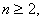working (each one individually) on the same problem. Denote by S1, S2 and S3 respectively the stages of imagination, visualization and ideas generation of the reasoning process. Denote also by a, b, c, d, and e the linguistic labels of very low, low, intermediate, high and very high success respectively of a person in each of the Si’s. Set U = {a, b, c, d, e}. We are going to attach to each stage Si of the reasoning process, i=1, 2, 3 , a fuzzy subset, Ai of U. For this, if nia, nib, nic, nid and nie denote the number of individuals that faced very low, low, intermediate, high and very high success at stage Si respectively, i=1,2,3, we define the membership function mAi for each x in U, as follows:In fact, if one wanted to apply probabilistic standards in measuring the degree of the individuals’ success at each stage of the process, then he/she should use the relative frequencies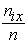. Nevertheless, such an action would be highly questionable, since the nix‘s are obtained with respect to the linguist labels of U, which are fuzzy expressions by themselves. Therefore the application of a fuzzy approach by using membership degrees instead of probabilities seems to be more suitable for this case. But, as it is well known, the membership function needed for such purposes is usually defined empirically in terms of logical or/and statistical data. In our case the above definition ofseems to be compatible with the common logic.

Then the fuzzy subset Ai of U corresponding to Si has the form:In order to represent all possible individuals’ profiles (overall states) during the reasoning process we consider a fuzzy relation, say R, in U3 of the form:For determining properly the membership function mR we give the following definition:

A profile s=(x, y, z), with x, y, z in U, is said to be well ordered if x corresponds to a degree of success equal or greater than y and y corresponds to a degree of success equal or greater than z.

For example, (c, c, a) is a well ordered profile, while (b, a, c) is not.

We define now the membership degree of a profile s to be mR(s) = m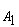(x)m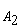(y)m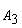(z) if s is well ordered, and 0 otherwise.

In fact, if for example the profile (b, a, c) possessed a nonzero membership degree, how it could be possible for a person, who has failed at the visualization stage, to perform satisfactorily at the stage of the ideas generation?

Next, for reasons of brevity, we shall write ms instead of mR(s). Then the probability ps of the profile s is defined in a way analogous to crisp data, i.e. byWe define also the possibility rs of s to be rs=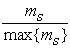where max{ms} denotes the maximal value of ms , for all s in U3. In other words the possibility of s expresses the “relative membership degree” of s with respect to max{ms}.

Assume further that one wants to study the combined results of behaviour of k different groups of people, k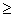2, during the reasoning process. For this, we introduce the fuzzy variables A1(t), A2(t) and A3(t) with t=1, 2,…, k. The values of these variables represent fuzzy subsets of U corresponding to the stages of the reasoning process for each of the k groups; e.g. A1(2) represents the fuzzy subset of U corresponding to the stage of imagination for the second group (t=2).

Obviously, in order to measure the degree of evidence of the combined results of the k groups, it is necessary to define the probability p(s) and the possibility r(s) of each profile s with respect to the membership degrees of s for all groups. For this reason we introduce the pseudo-frequenciesand we define the probability and possibility of a profile s by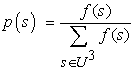andrespectively, where max{f(s)} denotes the maximal pseudo-frequency.

The same method could be applied when one wants to study the combined results of behaviour of a group during k different reasoning situations.

The above model gives, through the calculation of probabilities and possibilities of all individuals’ profiles, a quantitative/qualitative view of their realistic performance at all stages of the reasoning process.

### 3. Measures of Uncertainty

Within the domain of possibility theory uncertainty consists of strife (or discord), which expresses conflicts among the various sets of alternatives, and non-specificity (or imprecision), which indicates that some alternatives are left unspecified, i.e. it expresses conflicts among the sizes (cardinalities) of the various sets of alternatives (; p. 28).

Strife is measured by the function ST(r) on the ordered possibility distribution r: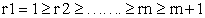of a group of a system’s entities defined by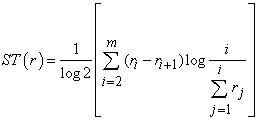, while non-specificity is measured by the function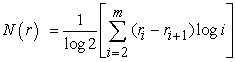.

The sumis a measure of the total possibilistic uncertainty for ordered possibility distributions. The lower is the value of T(r), which means greater reduction of the initially existing in the system uncertainty, the better the system’s performance.

Another fuzzy measure for assessing a system’s performance is the well known from classical probability and information theory Shannon’s entropy . For use in a fuzzy environment, this measure is expressed in terms of the Dempster-Shafer mathematical theory of evidence in the form:(, p. 20).

In the above formula n denotes the total number of the system’s entities involved in the corresponding process. The sum is divided by ln n (the natural logarithm of n) in order to be normalized. Thus H takes values in the real interval [0, 1]. The value of H measures the system’s total probabilistic uncertainty and the associated to it information. Similarly with the total possibilistic uncertainty, the lower is the final value of H, the better the system’s performance.

An advantage of adopting H as a measure of uncertainty instead of T(r) is that H is calculated directly from the membership degrees of all profiles s without being necessary to calculate their probabilities p(s). In contrast, the calculation of T(r) presupposes the calculation of the possibilities r(s ) of all profiles first. However, according to Shackle  and many other researchers after him, human reasoning can be formalized more adequately by possibility rather, than by probability theory. But, as we have seen in the previous section, the possibility is a kind of “relative probability”. In other words, the “philosophy” of possibility is not exactly the same with that of probability theory. Therefore, on comparing the effectiveness of two systems by these two measures, one may find non compatible results in boundary cases, where the systems’ performances are almost the same.

### 4. Classroom Experiments

In order to illustrate the use of our fuzzy model in practice we performed recently the following two experiments at the Graduate Technological Educational Institute (T. E. I.) of Patras, Greece [11, 12].

In the first experiment our subjects were 35 students of the School of Technological Applications, i.e. future engineers. A few days before the experiment an analysis of the scientific reasoning process (see introduction) was presented to the students in a two hours lecture, followed by a number of suitable examples. The following two problems with their relevant analyses were included among these examples:

Problem 1: We want to construct a channel to run water by folding across its longer side the two edges of an orthogonal metallic leaf having sides of length 20 cm and 32 cm, in such a way that they will be perpendicular to the other parts of the leaf. Assuming that the flow of the water is constant, how we can run the maximum possible quantity of the water?

Analysis of the problem:

IMAGINATION: The basic thing to realize is that the quantity of water to run through the channel depends on the area of the vertical cut of the channel.

VISUALIZATION (geometric configuration): Folding the two edges of the metallic leaf by length x across its longer side the vertical cut of the constructed channel is an orthogonal with sides x and 32-2x (Figure 3).

Figure 1. The vertical cut of the channel

IDEAS GENERATION: The area of the orthogonal has to be maximized.

The above idea leads to the following mathematical manipulation:

The area is equal to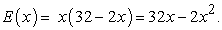Taking the derivative E΄(x) the equation E΄(x) = 32-4x = 0 gives that x = 8 cm. But E΄΄(x) = - 4 < 0, therefore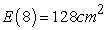is the maximum possible quantity of water to run through the channel.

Problem 2: The rate of increase of the population of a country is analogous to the number of its inhabitants. If the population is doubled in 50 years, in how many years it will be tripled?

Analysis of the problem:

IMAGINATION: The key concepts involved in the statement of this problem are the ‘analogy’ and the ‘rate of increase’ of the population. Therefore the crucial action for the solution of the problem is to establish the relation connecting these two concepts.

VISUALIZATION: The population P of the country is obviously a function of the time t, say P = P(t). In observing the increase of the population we must consider a starting point, where t = 0.

IDEAS GENERATION: The rate of increase of the population is expressed by the derivative P΄(t) and the existing analogy is expressed by P΄(t) = k P(t), with k a non negative integer. Therefore the solution of the problem is based on the solution of the above differential equation.

This suggests the following mathematical manipulation:

Separating the variables we can write, or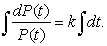Thus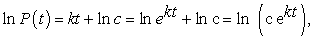or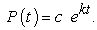For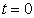we find that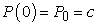and therefore we get that(1)

Further, according to the problem’s statement, we have that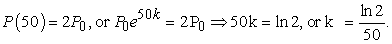Therefore (1) finally gives that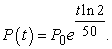If the population will be tripled after x years, then we’ll have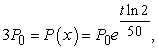orwhich gives that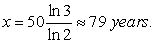In performing the experiment the following problem was given for solution to the students (time allowed 20 minutes):

Problem 3: Among all cylinders having a total surface of 180π m2, which one has the maximal volume?

Before starting the experiment we gave the proper instructions to students emphasizing, among the others, that we were interested for all their efforts (successful or not) during the reasoning process, and therefore they must keep records on their papers for all of them, at all stages of the process. In particular, we asked them to provide an analysis of the solution of the given problem analogous to the analyses presented for the above two examples.

Ranking the students’ papers by using the scale applied in section 3 (before the construction of Figure 1) we found that 15, 12 and 8 students had intermediate, high and very high success respectively at stage S1 of imagination. Therefore we obtained that n1a=n1b=0, n1c=15, n1d=12 and n1e=8. Thus, by the definition of the corresponding membership function given in the second section, S1 is represented by a fuzzy subset of U of the form:In the same way we represented the stages S2 and S3 as

fuzzy sets in U by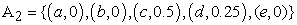and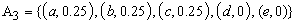respectively.

Next we calculated the membership degrees of the 53 (ordered samples with replacement of 3 objects taken from 5) in total possible students’ profiles as it is described in the second section (see column of ms(1) in Table 1). For example, for the profile s=(c, c, a) one finds that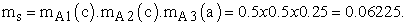It is straightforward now, using the corresponding formula given in section 3, to calculate in terms of the membership degrees the Shannon’s entropy for the student group, which is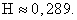Further, from the values of the column of ms(1) it turns out that the maximal membership degree of students’ profiles is 0.06225. Therefore the possibility of each s in U3 is given byOne, in order to be able to make the corresponding comparisons, could also calculate the probabilities of the students’ profiles using the formula for ps given in section 2. However, adopting the Shackle’s view (see section 3) we considered that the calculation of the probabilities is not necessary.

Calculating the possibilities of all profiles (see column of rs(1) in Table 1) one finds that the ordered possibility distribution for the student group is: r1=r2=1,r3=r4=r5=r6=r7=r8=0,5,r9=r10=r11=r12=r13=r14=0,258, r15=r16=……..=r125=0. Thus using a calculator we found thatandTherefore we finally obtained thatA few days later we performed the same experiment with a group of 50 students from the School of Management and Economics. Working as in the first experiment we found that: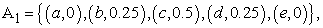and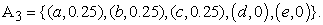Then we calculated the membership degrees of all possible profiles of the student group (column of ms (2) in Table 1) and the Shannon’s entropy, which is H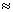0,312. Further, since the maximal membership degree is again 0.06225, the possibility of each s is given by the same formula as for the first group. The values of the possibilities of all profiles are given in column of rs(2) of Table 1.

Calculating the possibilities of all profiles (column of rs(2) in Table 1) one finds that the ordered possibility distribution of the second group is: r: r1 = r2 = 1, r3 = r4= r5 = r6 = r7 = r8 = 0,5 , r9 = r10 = r11 = r12 = r13 = 0,258, r14 = r15 =…….= r125=0. Then, working in the same way as above for the first group, one finds that T(r) = 0,432+2,179 = 2,611. Therefore, since 2,611<2,653, it turns out that the second group had in general a slightly better performance than the first one.

Notice that the values of the Shannon’s entropy found above for the two groups lead to the opposite conclusion (since 0,312>0,289), but this, as we have already explained in the third section, is not surprising in cases where the difference between the performances of the two groups is very small.

Finally, in order to study the combined results of the two groups’ performance we calculated the pseudo-frequencies f(s) = ms(1)+ms(2) and the combined possibilities of all profiles (see the last two columns of Table 1 as it has been described in section 2 of the present paper.

#### Table 1. Profiles with non zero membership degreesDownload asPowerPoint Slide

Veiw figureView current table in a new window

Notice that our model can be also used (in a simplified form) for the individual assessment of the students of each group. In this case a qualitative profile (x, y, z) with x, y, z in U is assigned to each student concerning his/her performance at each stage of the reasoning process. This type of assessment by reference to the profile related to each student defines in general a relation of partial order among students’ with respect to their total performance. For example, consider the student profiles of Table 1. Then the student possessing the profile (d, b, b) demonstrates a better performance than the student possessing the profile (b, b, b), or (c, b, a), or (d, a, a), etc. However, the student with profile (d, c, a) demonstrates a better performance at the stage of visualization than the student with profile (d, b, b), which demonstrates a better performance at the stage of generation of ideas, etc (for more details see ).

### 5. Conclusions and Discussion

The following conclusions can be drawn from the material presented in this paper:

Ÿ  The main stages of human reasoning for reaching the solution of a given problem involve imagination, visualization and idea generations. The above stages are helpful in understanding the individuals’ ‘ideal behaviour’ during the reasoning process. However, things in real situations are usually not happening like that, since human cognition utilizes in general concepts that are inherently graded and therefore fuzzy.

Ÿ  In this paper we made use of a fuzzy model for a better description of the reasoning process developed in earlier papers [11, 12]. This model gives, through the calculation of probabilities and possibilities of all possible individuals’ profiles, a quantitative/qualitative view of their behaviour during the process. Based on the above model and using well known measures of a system’s uncertainty we developed techniques of measuring the reasoning skills of a group of individuals. Our model can be also used (in a simplified form) for the individual assessment of the members of each group.

Ÿ  We also presented classroom experiments performed with student groups’ of T. E. I. of Patras, Greece illustrating our results in practice.

Here we must emphasize that the centroid method presented in earlier papers [11, 12] treats differently the idea of a system’s performance, than the two measures of uncertainty presented in this paper do. In fact, the weighted average plays the main role in the centroid method, i.e. the result of the system’s performance close to its ideal performance has much more weight than the one close to the lower end. In other words, while the measures of uncertainty are dealing with the average system’s performance, the centroid method is mostly looking at the quality of the performance. Consequently, some differences could appear in evaluating a system’s performance by these different approaches. Therefore, it is argued that a combined use of all these (3 in total) measures could help the user in finding the ideal profile of the system’s performance according to his/her personal criteria of goals.

Our plans for future research on the subject involve:

Ÿ  The possible extension of our fuzzy model for the description of other real life situations involving fuzziness and/or uncertainty.

Ÿ  Further experimental applications of our model in order to obtain more creditable statistical data.

### Acknowledgement

The heading of the Acknowledgment section and the References section must not be numbered.

### References

  Klir, G. J. & Folger, T. A., Fuzzy Sets, Uncertainty and Information, Prentice-Hall, London, 1988.In article  Klir G. J., “Principles of uncertainty: What are they? Why do we need them?” Fuzzy Sets and Systems, 74, 15-31, 1995.In article CrossRef  Sen, Z., Fuzzy Logic and Hydrological Modelling, Taylor & Francis Group, CRC Press, 2010.In article  Sen, Z., “Fuzzy philosophy of science and education”, Turkish Journal of Fuzzy Systems, 2 (2), 77-98, 2011.In article  Shackle, G. L. S., Decision, Order and Time in Human Affairs, Cambridge University Press, Cambridge, 1961.In article  Shannon, C. E., “A mathematical theory of communications”, Bell Systems Technical Journal, 27, 379-423 and 623-656, 1948.In article  Voskoglou, M. Gr., Stochastic and fuzzy models in Mathematics Education, Artificial Intelligence and Management, Lambert Academic Publishing, Saarbrucken, Germany, 2011 (for more details look at http://amzn.com./3846528218).In article  Voskoglou, M. Gr., “Fuzzy Logic and Uncertainty in Mathematics Education”, International Journal of Applications of Fuzzy Sets and Artificial Intelligence, 1, 45-64, 2011.In article  Voskoglou, M. Gr., “A study on Fuzzy Systems”, American Journal of Computational and Applied Mathematics, 2(5), 232-240, 2012.In article CrossRef  Voskoglou, M. Gr., “Assessing Students’ Individual Problem Solving Skills”, International Journal of Applications of Fuzzy Sets and Artificial Intelligence, 3, 39-49, 2013.In article  Voskoglou, M. Gr., “An Application of Fuzzy Sets to Scientific Thinking”, ICIT 2013: The 6th International Conference on Information Technology, May 8, 2013, available in the web at: http://sce.zui.edu.jo/icit13/images/Camera%20Ready/Artificial%20Intelligence/638_math.pdf.In article  Voskoglou, M. Gr & Subbotin, I. Ya, “Dealing with the Fuzziness of Human Reasoning”, International Journal of Applications of Fuzzy Sets and Artificial Intelligence, 3, 91-106, 2013.In article  Zadeh, L. A., “Fuzzy Sets”, Information and Control, 8, 338-353, 1965.In article CrossRef  Zadeh, L. A., “Fuzzy Algorithms”, Information and Control, 12, 94-102, 1968.In article CrossRef